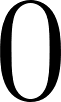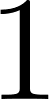Discrete Mathematics With Applications - 4 Edition - Chapter 1.2 - Problem 2
Register Now

Join StudySoup

Get Full Access to Discrete Mathematics With Applications - 4 Edition - Chapter 1.2 - Problem 29780495391326

# Write in words how to read each of the following out loud. a. {x R+ | 0 < x < 1} b. {x R

Discrete Mathematics with Applications | 4th Edition

Problem 2

Write in words how to read each of the following out loud.

a. $$\left\{x \in \mathbf{R}^{+} \mid 0<x<1\right\}$$

b. $$\{x \in \mathbf{R} \mid x \leq 0 \text { or } x \geq 1\}$$

c. $$\{n \in \mathbf{Z} \mid n \text { is a factor of } 6\}$$

d. $$\left\{n \in \mathbf{Z}^{+} \mid n \text { is a factor of } 6\right\}$$

Accepted Solution
Step-by-Step Solution:

Step 1 of 4

a)is an element of the set of positive real numbers,whereis greater thanand less than.

###### Chapter 1.2, Problem 2 is Solved

Step 2 of 4

Step 3 of 4

Unlock Textbook Solution